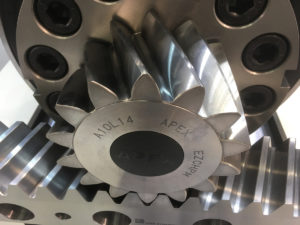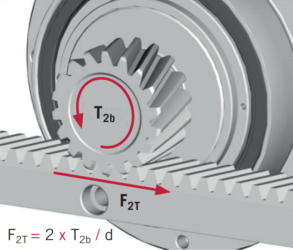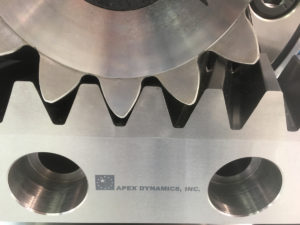Back to the overview

# Calculating rack and pinion, how do you do that?Calculate a rack and pinion drive, how do you do that?
If you immerse yourself in this you will be confused with terms and formulas that make the situation very complex. That is why we try to explain to you in this article how you can do such calculation the easiest.

An important principle is that you realize that the calculation and selection (rack, pinion, gearbox and motor) is done by trial-and-error: you have a good chance that you have to do the calculations again with other parameters, such as the diameter of the pinion or the quality (read in this case: hardness) of the rack.

## Calculate rack and pinion: important definitions

To make it clear we give you some important definitions:

•Tangential force or feed force.
This is the force [in N] needed to deliver the linear movement profile.
• Torque.
This is what the pinion sees and is simply tangential force * arm (radius pinion) [in Nm]. In this case F2T = 2 * T2B / d.
• Safety factor.
Apex recommends a safety factor of at least 2 for horizontal and 3 for vertical drives.
• Friction coefficient.
How heavy – or light – does the system run? A widely used value is 0.1 or 0.15.
• External forces.
For example: is the system used to push products? Then this force should be added to the tangential force.

## Points of attention for the selection of rack and pinionSometimes we confuse matters or we have assumptions that are not entirely correct. This makes it only harder to calculate the rack and pinion. Therefore keep the following in mind:

• A pinion of about 20 teeth is mathematically the optimum in terms of tangential force and system backlash. A larger pinion provides more backlash, a smaller pinion can transmit lower torques and has a higher wear.
• A larger module does NOT have to mean that the tangential force is higher! A rack module 2 in quality 5 can transmit a higher tangential force than module 3 in quality 8!
• The quality of a rack is not only the deviation per meter, but also the treatment and hardness. For example, quality 8 has a better price than quality 10!
• Backlash is caused by the interaction of ALL components. It makes no sense to take a very-low-backlash gearbox with, for example, a larger pinion or a lower quality rack.
• The tolerances of the racks and pinions are NOT standardized, in practice we often see deviations. For example, by giving the total deviation per 300 mm instead of per 1000 mm. The first 12 pages of our catalog are about the tolerances of OUR products, how we have defined them and what the value is. From linear deviation to hardness and straightness, ALL tolerances can be found.
• For a silent and low-backlash drive with a long service life, it is recommended to use a pinion and rack from 1 supplier. The tolerances, especially with helical teeth, are very important!

## Calculate rack and pinion: calculation tool

With the table below you can perform the calculations:

 Application Horizontal movement Vertical movement Unit Application settings Total weight load m kg kg Speed v m/s m/s Time acceleration ta s s Gravity g /s2 /s2 Friction coefficient µ – – Pitch circle pinion d mm mm Other forces F N N Safety factor SB – – Formulas ∝ = V / t∝ (m/s2) ∝ = V / t∝ (m/s2) Tangential force FN FN = M * g * µ + M * a + F (N) FN = M * g * µ + M * a + F (N) Torque TN TN = (FN * d) / 2000 (Nm) TN = (FN * d) / 2000 (Nm) Design torque TNV TNV = TN * SB (Nm) TNV = TN * SB (Nm) Max. speed pinion NV NV = (V * 19100) / d (rpm) NV = (V * 19100) / d (rpm)

The tables can be found in our catalog, after the pinions sector, here the F2T and T2B are given for the different pinions and racks. Based on this and the servomotor specifications you can calculate the gearbox.

Because the rack and pinion is often trial-and-error, we like to take these calculations out off your hands. When we receive the correct application information, our customers – often within and day – receive a calculation sheet, quotation and STP drawings of all items: rack, pinion and gearbox.

This sheet is the basis for the choosen components and can be part of the technical construction file. We can also give an indication for the backlash of the system, so that it can be even optimized.